# A Macro-Mathematical Model for the Observed Value of Digital BlockChain Networks

(A slightly more detailed version of this article was published in the Electronic Commerce Research and Applications, Vol 24, July-Aug 2017 ed.)

Digital blockchain networks have been around for nearly a decade. Digital contracts and cryptocurrency transactions between users on a blockchains, for instance, result in a de-facto network of those users connected in digital space. Due to the fact that these networks are online and published on public ledgers, the blockchain, data on the network is more readily available than most prior networks. This allows us to analyze these networks in unprecedented ways, and derive macro-mathematical relationships or models between entities on the network.

In this article, we report on one such analysis, to determine if the observed value of the networks, as measured by how much users appear willing to pay to participate in the network, can be modeled as a function of the number of users on it. This relationship, where it exists is known as the network effect.

Network effect basically means that the value of a network grows as the number of users on the network. A network model presents a macro-mathematical relationship between the value of the network and users on the network. Considering the example of a new phone network that has only two users initially, the allure of the network to a third user would be the need to communicate with the currently existing two users on the network. That demand would be related to the known circle of the two initial users. As more users join the network, the number of other users they can reach increases, as does the demand to join the network, increasing the network’s value to even more users, in general

Robert Metcalfe, in inventing the Ethernet, which helped usher in the growth of the Internet as a global network about four decades ago proposed a relationship for the value of a network in relationship to the size of the network . He stated that the value of the network is proportional to the number of connections of users that can be made on the network, which computed to N(N-1). As N becomes very large this approximates to a square of the nodes on the network (VNN.) Metcalfe’s law has the form:

Other network models include those of Sarnoff  formulated for a network of viewers of a television network, Odlyko , and Reed . The relationship proposed for these models are shown below.

These relationships had been hitherto difficult to verify due to the fact that data needed for that verification is difficult to obtain as well as how to measure and define network value. However, several works recently on social media networks have seemed to validate Metcalfe’s relationship when applied to large social networks including Facebook , and later to Tencent , China’s largest social network.

We extracted the necessary data from three blockchain networks — Bitcoin, Ethereum, and Dash — and analyzed the data to determine if they demonstrate network effects and could be modeled by one of the foregoing models, or if we could derive a better model for their observed growth.

For blockchains, there are several sub-networks in the system. The first is the network of end users connected over the blockchain and holding assets that can be exchanged or transacted among each other on the network. The second is the network of entities and computers that maintain the blockchain and process transactions on the network. A third is the network of connected merchants on that network, provided the digital asset is a medium of exchange for merchant goods or services; or of developers and companies developing products on the network, if the network allows creation of third party products or instruments. It is likely that the value of the network would have a positive mathematical relationship to each of these sub-networks. Here, the focus was on deriving relationships between the network entities as it relates to the number of end users on the network. The rate of growth in time of some of the blockchain networks is also compared to the netoid growth function. Based on an initial review, only Metclafe’s law came close to modeling the exponential growth in users and value of the networks analyzed and so was the focus of the current study. An alternative model for both the growth function as well as the value of the network is also proposed and compared with Metcalfe’s law.

Modeling the Growth and Value of Selected Blockchain End User Networks

The blockchains selected were all cryptocurrencies since the price and capitalization of the networks are available and published daily, in fact every second. So are several interesting relationships such as the number of unique IP addresses participating on the network, volume of transactions, and number of wallets, a denomination of user accounts on the network. The following are the parameters of this analysis.

1. The value of the network was modeled by the price of the digital currency established by the network. The price and capitalization have a direct relationship so either could be used without affecting the form of the model.

2. The number of end users was selected as the number of unique addresses participating on the network per day. This is in lieu of obtaining the actual number of active users on the network, which is difficult to decipher due to the privacy of account ownership, and since a user can establish several accounts, or wallets, in participating on the network. The assumption is that given a large number of participating users, the ratio of the number of actual users to the number of unique IP address would be roughly consistent.

3. The actual curve of price on the network is assumed to consist of bubbles and bursts — noise related to medium to short term events that need to be filtered out in order to obtain the true model of growth and value of the network. This is slightly similar to filtering of the smaller turbulent signals or length scales in fluid dynamics to derive the major length scales in a fluid flow. The daily number of unique address was also filtered to remove the statistical noise from the data.

4. The growth of the network is expected to begin from the point when critical mass is reached. The critical mass is a threshold number of users from which point the network begins to grow, or becomes viral. Below this number, the value of the network remains roughly stagnant and close to its initial starting value. The growth of the network is also expected to have a deceleration point where the network begins to reach saturation, either due to technical limits or due to having reached much of its reachable base of users.

A few blockchains were selected that have at least two year’s worth of data. This includes bitcoin, ethereum, and dash.

The Growth Function

This is a model for the growth of the network in time. The netoid function proposed Metcalfe, is in the form of an ∫, with an initial exponential rapid growth, and later as the network reaches saturation a similar exponential deceleration with similar growth and deceleration rate.

The netoid takes the form of a sigmoid function as follows:

Where N the total number of users asymptotically goes to p at t tends to →∞. tm is the center of the sigmoid where the number of users is assumed to be at peak growth rate and N = p/2. v is the virality of the network and represents how fast the network is growing.

None of the blockchains we examined have yet reached a point of stagnating value so we would only be able to model the initial growth portion of the network. In addition, there no basis to expect that the parameter, v will also govern the deceleration rate of the network. Consequently, the growth models examined here will focus on the initial growth section of the network following when critical mass has been achieved.

Rationale for the Exponential Growth Relationship Inherent in the Netoid Function

In this section, an attempt at a rationale for the exponential relationship in the netoid is presented. Due to the fact that the assets in review in this paper have very little actual use currently (they are not really used much as a transactional medium for payment systems, nor even significantly as a payment rail), it may be reasonable to interpret their adoption to be based on their perceived future potential. If that potential or value proposition is presented to a selected number of people, the expectation would be that the same ratio in that set would convert into acquiring the assets. That group would then communicate that same value proposition to their own social network of friends and acquaintances, and on. Therefore, the basic adoption process might not be dissimilar from that of a social network. Then if N is the number of people adopting the assets at time t, at time t + Dt the number would be proportional to the number of people holding the assets currently. This can be written as

That equation has solutions in the form:

Where v is the exponential growth rate, and N0 is the initial value at the point where the growth begins (on achieving critical mass.)

A New Value-Size Function for Blockchain User Networks

A model for the value of the network in relation to the number of unique addresses participating in the network utilizing an exponential function was also derived and compared with Metcalfe’s law. The new model takes the form

The values of C, l, and m were determined from the fit to the equation. The filter applied to N is a 30 day average filter which takes the form:

Note that h = 0 would be a 30 day backward moving average and would result in a lagging N in relation to the value function. h was chosen to weight the filter evenly about the point at i for the exponential function, with its effect shown in the close fit between the filtered and actual quantity in the figure below.

Data Sources

The data on daily close price and unique addresses participating addresses on the Bitcoin network, Ethereum, and Dash, are available from the blockchain, and were obtained from blockchain.info, etherscan.io, and bitinfocharts.com.

Comparisons of the Models and Actual Growth Data of Selected Blockchain Assets

The figure below shows the comparison of the netoid function with actual data for Bitcoin going back nearly to its advent in 2009 up until June 2017.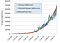Netoid Function fit for the Bitcoin Network. p = 1,500,000, v = 0.002, tm = 3200, and RMSD = 6%

It may be noted that based on this model, tm, the median time where the adoption rate is maximum, would be reached in October 2017, and that the network is already almost reaching about half of p. In addition, while the prior agreement between the model and the growth in the number of unique addresses participating on the network has been good, there are known technical issues on the network that remains unresolved at this point that could influence the future growth of the network.

The figure below is obtained on fitting Metcalfe’s law and the new model to the daily unique users.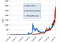Metcalfe’s Law and the New Model compared with actual daily price on the Bitcoin Network

The value of MetCalfe’s growth amplitude parameter, k, for the model was 4 x 10–9. For the new function, m = 0.5, l = 0.01, and C = 1. The RMSD of the models compared to the live data is 205, and 209 respectively for Metcalfe’s law and the proposed function or about 7.6% and 7.7% respectively compared to the close price at the last data point. However, on focusing on the recent trend, the new function appears to slightly better model the daily values as shown below.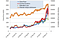Close up view of Metcalfe’s Law and the new Network Model Compared to Actual Price Values on the Bitcoin Network

The figure below shows the netoid plot for the ethereum network.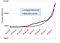Netoid Function fit for the Ethereum Network. p = 14,000,000, v = 0.01, and tm = 1120, and RMSD = 8%

It is also interesting to note that the growth rate of this network, as currently observed is about five times that of the Bitcoin network, and the number of unique addresses participating on the network has a higher ceiling. In addition, the maximum growth time, tm, the point of maximum adoption rate, would be attained some time in August 2018 assuming the model continues to hold. The model of the network value with the number of daily unique addresses is shown below.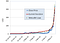Metcalfe’s Law and the New Model compared with daily ETH price on the Ethereum Network

For the Ethereum network, the value of MetCalfe’s growth amplitude parameter, k, for the model was 2.8 x 10–7. For the new function, m = 0.5, l = 0.02, and C = 6. The RMSD of the models compared to the live data is 4.3% for Metcalfe’s Odlyko’s and 4.1% for the new model compared to the close price at the last data point.

Similar plots were made for the Dash network with the values of k =3 x 10–7 for the Metcalfe’s growth parameter. For the new function, m = 0.5, l = 0.033, and C = 1. The RMSD for both models were 13% and 12%, respectively. Plots of this and additional discussion on the methodology and the results will be available in an upcoming computational journal.

For the new function, based on the data, since m = 0.5 in all the cases observed, the form of the proposed model for the growth of the network as a function of the number of unique addresses participating actively on the network is:

the observed growth rate is exponentially proportional to the root of the number of active users. The form of the proposed model allows some interesting value based comparisons of each network, beyond what the Metcalfe’s law provides. It can be adaptively fitted to a network based on the intrinsic characteristics of the network, since it contains a separate growth multiplier of the number of active users and a linear growth multiplier C similar to that of Metcalfe’s law. In addition, the form of the proposed model does not result in a pre-ordained growth exponent in the manner that the square of N does.

As a side note, it would be interesting to see if this new model could be applicable even to fiat currency networks, with the expectation that fiat currencies enjoying the most daily use would prove to be more valuable and exhibit similar network growth effects, if the monetary supply side policies can somehow be quantified, removed or also incorporated into the model.

# Analysis of Critical Mass

The prior figures for the daily number of unique users shows visually that the growth and value of the network remained flat from inception up until a point when the growth in the number of users became exponential . Based on this, two conditions are introduced as potential signals or conditions for network effects:

The number of daily unique addresses has to reach a threshold. Its derivative also needs to reach a critical threshold. More work would need to be done to confirm these conditions using data from more such networks. However, based on these criteria, for the Bitcoin and Ethereum networks, the critical parameters appear to be about 100, and 20,000, respectively.

# Long Term Persistence of the Networks

In as much as the value of the network correlates with the daily number of users actively participating in it, that value can likely prove to be as fleeting as the ease with which those users could move to a different network or cease to participate in any of the networks. The assets designated for use on the networks are quite fungible and can be easily moved to other. That fungibility also means that users could create new addresses on different networks and move their assets there easily or simply just pull their assets out. In short, the ease with which users can move from the blockchain networks of the types studied here exceeds that of networks such as social media where the user might have cherished items including pictures, conversations, social contacts, and other historical items that might not be as easy to move. Consequently, care must be taken in recognizing that the value is correlated to the daily active unique addresses rather than the total number of unique addresses in time.

Any form of lock-in of the value of the network to any extent may turn out to be more related to some of its other sub-networks mentioned earlier. This includes how many businesses or merchants are participating in the network, how many developers have invested time and resources in creating products on the network.

Finally, it should be noted that since the number of daily unique users on the network is likely about as much a lagging indicator as the price of the assets denominated on the network, the purpose of these models is not intended as a speculative tool, but rather a means of modeling or understanding the characteristics of transactional public networks. Based on the agreement observed between the models presented here, hopefully this article will greatly further that objective.

Please email any request for the frozen spreadsheet used for this analysis.

If you would like to see more analysis like this or for more analysts to potentially perform similar for some of the other networks, then please use the ❤ heart below to recommend this article so that others can see it.

References

 Metcalfe B. Metcalfe’s law after 40 years of Ethernet. IEEE Computer, 2013, 46(12): 26–31.

 Van Hove L. Metcalfe’s law: Not so wrong after all. NETNOMICS: Economic Research and Electronic Networking,

2014, 15(1): 1–8.

 Zhang XZ, Liu JJ, Xu ZW. Tencent and Facebook data validate Metcalfe’s law. Journal of Computer Science and Technology 30(2): 2015, 246–251.

 Swann G M. The functional form of network effects. Information Economics and Policy, 2002, 14(3): 417–429.

 Briscoe B, Odlyzko A, Tilly B. Metcalfe’s law is wrong. IEEE Spectrum, 2006, 43(7): 34–39.

 Reed D P. That Sneaky exponential — Beyond Metcalfe’s law to the power of community building. Context Magazine, 1999, 2(1). http://www.reed.com/dpr/locus/-gfn/reedslaw.html, Jan. 2015.

 Data on the Bitcoin Network. https://blockchain.info/charts, Retrieved June 6 2017.

 Data on the Ethereum Network. https://etherscan.io/charts, Retrieved June 6 2017.

 Data on the Dash Network. https://bitinfocharts.com/dash, Retrieved June 6 2017.

 Ball P. Critical Mass: How One Thing Leads to Another. Published by Farrar, Straus and Giroux 1st Edition, ISBN-10: 0374281254, 2014.

Written by

Written by

## More From Medium

Welcome to a place where words matter. On Medium, smart voices and original ideas take center stage - with no ads in sight. Watch
Follow all the topics you care about, and we’ll deliver the best stories for you to your homepage and inbox. Explore
Get unlimited access to the best stories on Medium — and support writers while you’re at it. Just \$5/month. Upgrade